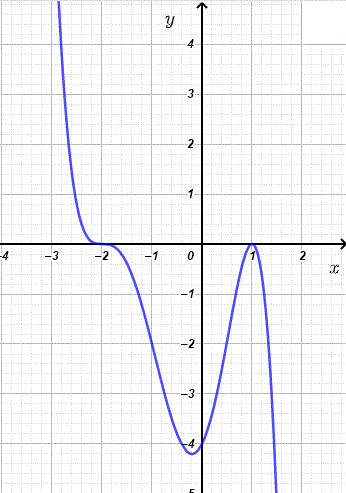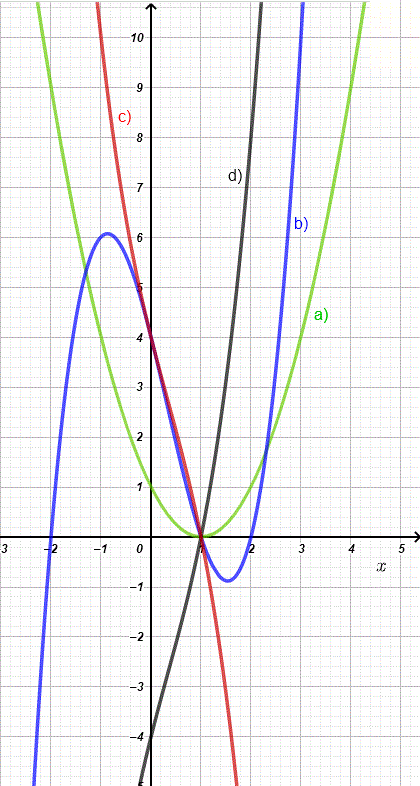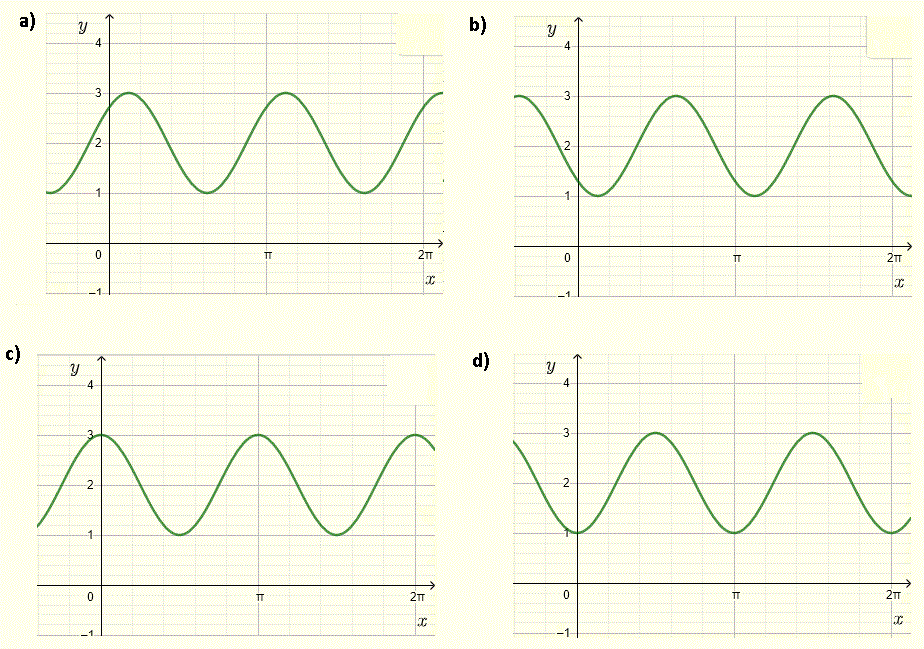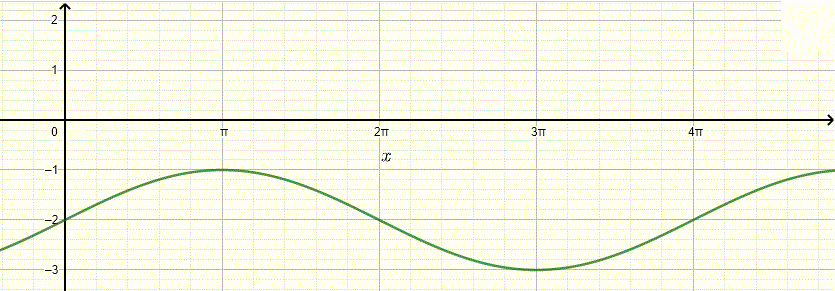# Algebra 2 Problems

A set of algebra 2 problems with their detailed solutions to self test and diagnose your background and review and gain deep understanding on the following topics:
1. Complex Numbers
1. Adding and Subtracting Complex Numbers
2. Conjugate of Complex Numbers
3. Multiplying and Dividing Complex Numbers
1. Solving Quadratic Equations with Complex Solutions
2. Number and Nature of Solutions of Quadratic Equations
3. Functions
1. Evaluate a function
2. Domain and Range of a function
3. Operations on functions
4. One-to-one functions
5. Inverse of a function
6. Absolute Value function
7. Even and Odd functions
8. Transformations of functions
9. Piecewise functions
10. Rate of Change of a Function
4. Polynomials Functions
1. Dividing Polynomials
2. Factoring Polynomials
3. Factoring Polynomials Using Special Polynomials
4. Solving Polynomial Equations
5. Solving Polynomial Inequalities
6. Graphing Polynomials Functions
5. Rational Expressions, Equations, Inequalities and Functions
1. Simplifying Rational Expressions
2. Solving Rational Equations
3. Solving Rational Inequalities
4. Graphing Rational Functions
6. Trigonometry and Trigonometric Functions
1. Converting Degrees into Radians and Vice Versa
2. Evaluating Exact Values of Trigonometric Ratios
3. Using Trigonometric Identities to Simplify Trigometric Expressions
4. Solving Trigonometric Equations
5. Graphing Trigonometric Functions
7. Logarithmic and Exponential Functions
1. Simplifying Exponnential Expressions
2. Using Relationship Between Logarithmic and Exponential Expressions
3. Simplifying Logarithmic Expressions
4. Solving Logarithmic and Exponential Equations
5. Graphing Logarithmic and Exponential Functions

## Algebra 2 problems with detailed solutions

1. ### Complex Numbers

2. Problem 1-1
Let z = 2 - 3 i where i is the imaginary unit. Evaluate z z* , where z* is the conjugate of z , and write the answer in standard form.

Problem 1-2
Evaluate and write in standard form $$\dfrac{1-i}{2-i}$$ , where i is the imaginary unit.

4. Problem 2-1
Find all solutions of the equation $$x(x + 3) = - 5$$.

Problem 2-2
Find all values of the parameter m for which the equation $$-2 x^2 + m x = 2 m$$ has complex solutions.

5. ### Functions

6. Problem 3-1
Let $$f(x) = - x^2 + 3(x - 1)$$. Evaluate and simplify $$f(a-1)$$.

Problem 3-2
Write, in interval notation, the domain of function $$f$$ given by $$f(x) = \sqrt{x^2-16}$$.

Problem 3-3
Find and write, in interval notation, the range of function $$f$$ given by $$f(x) = - x^2 - 2x + 6$$.

Problem 3-4
Let $$f(x) = \sqrt{x - 2}$$ and $$g(x) = x^2 + 2$$; evaluate $$(f_o g)(a - 1)$$ for $$a \lt 1$$.

Problem 3-5
Which of the following is a one-to-one function?(There may be more than one answer).
a) $$f(x) = - 2$$     b) $$g(x) = \ln(x^2 - 1)$$     c) $$h(x) = |x| + 2$$     d) $$j(x) = 1/x + 2$$     e) $$k(x) = \sin(x) + 2$$     f) $$l(x) = ln(x - 1) + 1$$

Problem 3-6
What is the inverse of function f given by $$f(x) = \dfrac{-x+2}{x-1}$$?

Problem 3-7
Classify the following functions as even, odd or neither.
a) $$f(x) = - x^3$$     b) $$g(x) = |x|+ 2$$     c) h(x) = $$\ln(x - 1)$$

Problem 3-8
Function $$f$$ has one zero only at $$x = -2$$. What is the zero of the function $$2f(2x - 5)$$?

Problem 3-9
Which of the following piecewise functions has the graph shown below?
a) $$f(x) = \begin{cases} x^2 & \text{if} \; x \ge 0 \\ 2 & \text{if} \; -2 \lt x \lt 0\\ - x + 1& \text{if} \; x \le -2 \end{cases}$$     b) $$g(x) = \begin{cases} x^2 & \text{if} \; x \gt 0 \\ 2 & \text{if} \; -2 \lt x \le 0\\ - x + 1& \text{if} \; x \le -2 \end{cases}$$     c) $$h(x) = \begin{cases} x^2 & \text{if} \; x \gt 0 \\ 2 & \text{if} \; -2 \lt x \lt 0\\ - x + 1 & \text{if} \; x \lt -2 \end{cases}$$Problem 3-10
Calculate the average rate of change of function $$f(x) = \dfrac{1}{x}$$ as x changes from $$x = a$$ to $$x = a + h$$.

7. ### Polynomials

8. Problem 4-1
Find the quotient and the remainder of the division $$\dfrac{-x^4+2x^3-x^2+5}{x^2-2}$$.

Problem 4-2
Find $$k$$ so that the remainder of the division $$\dfrac{4 x^2+2x-3}{2 x + k}$$ is equal to $$-1$$?

Problem 4-3
$$(x - 2)$$ is one of the factors of $$p(x) = -2x^4-8x^3+2x^2+32x+24$$. Factor $$p$$ completely.

Problem 4-4
Factor $$16 x^4 - 81$$ completely.

Problem 4-5
Find all solutions to the equation $$(x - 3)(x^2 - 4) = (- x + 3)(x^2 + 2x)$$

Problem 4-6
Solve the inequality $$(x + 2)(x^2-4x-5) \ge (-x - 2)(x+1)(x-3)$$

Problem 4-7
The graph of a polynomial function is shown below. Which of the following functions can possibly have this graph?
a) $$y = -(x+2)^5(x-1)^2$$     b) $$y = 0.5(x+2)^3(x-1)^2$$     c) $$y = -0.5(x+2)^3 (x-1)^2$$     d) $$y = -(x+2)^3(x-1)^2$$Problem 4-8
Which of the following graphs could possibly be that of the function f given by $$f(x) = k (x - 1)(x^2 + 4)$$ where k is a negative constant? Find k if possible.9. ### Rational Expressions, Equations, Inequalities and Functions

Problem 5-1
Write as a single rational expression: $$\dfrac{x^2+3x-5}{(x-1)(x+2)} - \dfrac{2}{x+2} - 1$$.

Problem 5-2
Solve the equation: $$\dfrac{- x^2+5}{x-1} = \dfrac{x-2}{x+2} - 4$$.

Problem 5-3
Solve the inequality: $$\dfrac{1}{x-1}+\dfrac{1}{x+1} \ge \dfrac{3}{x^2-1}$$.

Problem 5-4
Find the horizontal and vertical asymptotes of the function: $$y = \dfrac{3x^2}{5 x^2 - 2 x - 7} + 2$$.

Problem 5-5
Which of the following rational functions has an oblique asymptote? Find the point of intersection of the oblique asymptote with the function.
a) $$y = -\dfrac{x-1}{x^2+2}$$     b) $$y = -\dfrac{x^4-1}{x^2+2}$$     c) $$y = -\dfrac{x^3 + 2x ^ 2 -1}{x^2- 2}$$     d) $$y = -\dfrac{x^2-1}{x^2+2}$$

Problem 5-6
Which of the following graphs could be that of function $$f(x) = \dfrac{2x-2}{x-1}$$?10. ### Trigonometry and Trigonometric Functions

11. Problem 6-1
A rotating wheel completes 1000 rotations per minute. Determine the angular speed of the wheel in radians per second.

Problem 6-2
Determine the exact value of $$sec(-11\pi/3)$$.

Problem 6-3
Convert 1200� in radians giving the exact value.

Problem 6-4
Convert $$\dfrac{-7\pi}{9}$$ in degrees giving the exact value.

Problem 6-5
What is the range and the period of the the function $$f(x) = -2\sin(-0.5(x - \pi/5)) - 6$$?

Problem 6-6
Which of the following graphs could be that of function given by: $$y = - \cos(2x - \pi/4) + 2$$?Problem 6-7
Find a possible equation of the form $$y = a \sin(b x + c) + d$$ for the graph shown below.(there are many possible solutions)Problem 6-8
Find the smallest positive value of x, in radians, such that $$- 4 \cos (2x - \pi/4) + 1 = 3$$

Problem 6-9
Simplify the expression: $$\dfrac{\cot(x)\sin(x) + \cos(x) \sin^2(x)+\cos^3(x)}{\cos(x)}$$

12. ### Logarithmic and Exponential Functions

13. Problem 7-1
Simplify the expression $$\dfrac{4x^2 y^8}{8 x^3 y^5}$$ using positive exponents in the final answer.

Problem 7-2
Evaluate the expression $$\dfrac{3^{1/3} 9^{1/3}}{4^{1/2}}$$.

Problem 7-3
Rewrite the expression $$\log_b(2x - 4) = c$$ in exponential form.

Problem 7-4
Simplify the expressiomn: $$\log_a(9) \cdot \log_3(a^2)$$

Problem 7-5
Solve the equation $$\log(x + 1) - log(x - 1) = 2 \log(x + 1)$$.

Problem 7-6
Solve the equation $$e^{2x} + e^x = 6$$.

Problem 7-7
What is the horizontal asymptote of the graph of $$f(x) = 2 ( - 2 - e^{x-1})$$?

Problem 7-8
What is the vertical asymptote of the graph of $$f(x) = log(2x - 6) + 3$$?

Problem 7-9
Match the given functions with the graph shown below?
A) $$y = 2 - 0.5^{2x-1}$$     B) $$y = 0.5^{2x-1}$$     C) $$y = 2 - 0.5^{-2x+1}$$     D) $$y = 0.5^{-2x+1}$$Problem 7-10
Match the given functions with the graph shown below?
A) $$y = 2+ln(x-2)$$     B) $$y=-log_2(x+1)-1$$     C) $$y = -ln(-x)$$     D) $$y = y=-log_3(x+1)-1$$